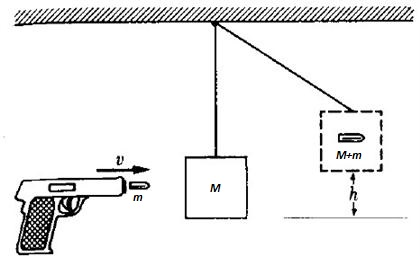# Calculate the bullet speed

One way of measuring a projectile's speed is by using a ballistic pendulum, as shown below.This pendulum is a piece of wood of mass $M$, suspended by a fine, lightweight wire. Initially, the pendulum is at rest. A bullet of mass $m$ horizontally strikes the piece of wood and gets stuck in it, causing the pendulum to lift up at height $h$.

If the mass of the pendulum is $M=4\text{ kg}$, the bullet's mass is $m=9.7 \text{ g}$, and after impact they lift up to $h=19\text{ cm}$, what is the initial speed (in $\text{m/s}$) of the bullet (to the nearest integer)?


Details and Assumptions:

• The gravitational acceleration is $g=9.8\text{ m/s}^2$.
×